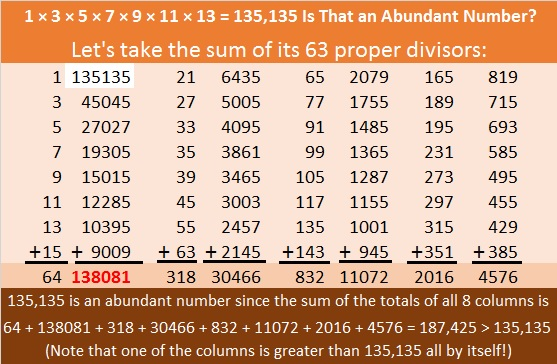There’s Something Odd about the Number 945

945 = 1 × 3 × 5 × 7 × 9

The sum of the proper divisors of a number determines if the number is abundant, deficient, or perfect. If the sum is greater than the number, the number is abundant. If the sum is less than the number, the number is deficient. If the sum is equal to the number, the number is perfect.

What is a proper divisor? All the factors of a number except itself. Proper divisors are ALMOST the same thing as proper factors. (The number 1 is always a proper divisor, but NEVER a proper factor.)

The first 25 abundant numbers are 12, 18, 20, 24, 30, 36, 40, 42, 48, 54, 56, 60, 66, 70, 72, 78, 80, 84, 88, 90, 96, 100, 102, 104, and 108. Notice that all those numbers are even.

OEIS informs us that 945 is the 232nd abundant number. The first 231 abundant numbers are all even numbers.Wow, 945 is the smallest ODD abundant number. OEIS also lists the first 31 odd abundant numbers. Every one of the first 31 is divisible by 3 and ends with a 5, but if you scroll down the page you’ll see some that aren’t divisible by 3 or aren’t divisible by 5.

Since 1 × 3 × 5 × 7 × 9 = 945 is the smallest number on the list, you may be wondering about some other numbers:
1 × 3 × 5 × 7 × 9 × 11 = 10,395 made the list.
1 × 3 × 5 × 7 × 9 × 11 × 13 = 135,135 which is too big to be one of the first 31 odd abundant numbers. I was curious if it is also an abundant number, so I found its proper divisors and added them up:945 is also the hypotenuse of a Pythagorean triple:
567-756-945 which is (3-4-5) times 189

945 looks interesting in a few other bases:
1661 in BASE 8 because 1(8³) + 6(8²) + 6(8¹) + 1(8⁰) = 945
RR in BASE 34 (R is 27 base 10), because 27(34¹) + 27(34⁰) = 27(35) = 945
R0 in BASE 35 because 27(35) + 0(1) = 945

• 945 is a composite number.
• Prime factorization: 945 = 3 × 3 × 3 × 5 × 7, which can be written 945 = 3³ × 5 × 7
• The exponents in the prime factorization are 3, 1, and 1. Adding one to each and multiplying we get (3 + 1)(1 + 1)(1 + 1) = 4 × 2 × 2 = 16. Therefore 945 has exactly 16 factors.
• Factors of 945: 1, 3, 5, 7, 9, 15, 21, 27, 35, 45, 63, 105, 135, 189, 315, 945
• Factor pairs: 945 = 1 × 945, 3 × 315, 5 × 189, 7 × 135, 9 × 105, 15 × 63, 21 × 45, or 27 × 35
• Taking the factor pair with the largest square number factor, we get √945 = (√9)(√105) = 3√105 ≈ 30.74085